# [on site] The Process of PID and IV Creation continued

#### X-Act

##### np: Biffy Clyro - Shock Shock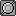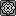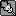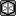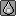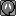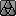6. More Things affected by the PID

6.1 How the Game Makes a Pokemon Shiny

Very rarely, you might encounter a Pokemon having a different coloration than usual. This is called a shiny Pokemon. The game’s criterion for making a shiny Pokemon depends in part on its PID, but also depends on two other numbers.

One of these numbers is visible in the game. It is called the Trainer ID. This is the number you have when you look at your Trainer card, and is a number between 0 and 65535 (between  and [FFFF] in hexadecimal). The other number is also a number between 0 and 65535 but is unfortunately invisible to you. It is called the Secret ID. Currently, there’s no way you can know your Secret ID without looking at your save file or without using cheat codes.

So how does the game determine that a Pokemon is shiny? Here’s how.

Split the PID in two 16-bit numbers. That is, if the PID was [465DB901], it would split it in two as [465D] and [B901]. We shall call the first 4 hexadecimal digits the HID (high ID) and the last 4 hexadecimal digits the LID (Low ID). Now convert the HID, LID, Trainer ID (which we shall denote by TID for short) and Secret ID (denoted by SID for short) to binary, and compare the first 13 bits of all four, like so:

Code:
``````HID: [U]x[/U]xxxxxxxxxxxx|xxx
LID: [U]x[/U]xxxxxxxxxxxx|xxx
TID: [U]x[/U]xxxxxxxxxxxx|xxx
SID: [U]x[/U]xxxxxxxxxxxx|xxx``````
Here we’re counting the number of 1's in the first bit of the HID, LID, TID and SID. If there are either 1 or 3 ones among those bits, the Pokemon is automatically not shiny. If there aren’t, the game counts the second bits. Again, if there are either 1 or 3 ones among those bits, the Pokemon is automatically not shiny. The game checks the bits of the HID, LID, TID and SID up to their thirteenth one, and all of them would need to have 0, 2 or 4 ones for the Pokemon to be shiny! This is a one in 8192 (2 to the power of 13) chance of happening.

Let’s have an example. Suppose the Trainer ID of the player is 43288, or [A918] in hexadecimal, and the Secret ID is 6075, or [17BB] in hexadecimal. Suppose also that the PID of the Pokemon is [B58F0B2A]. We split the PID in two, the HID [B58F] and the LID [0B2A], and convert the HID, LID, Trainer ID and Secret ID to binary. Thus we would have:

Code:
``````HID: 1011010110001|111
LID: 0000101100101|010
TID: 1010100100011|000
SID: 0001011110111|011``````
We now count the number of bits in the first bit, second bit, third bit ... up to the thirteenth bit of each of these four 16-bit numbers.

Code:
`````` HID: 1011010110001|111
LID: 0000101100101|010
TID: 1010100100011|000
SID: 0001011110111|011
Ones: 2022222420224``````
Since counting the ones of each bit up to the thirteenth one in turn never yields a number that is not 1 or 3, the Pokemon will be shiny!

6.2 How to determine whether Wurmple evolves into Silcoon or into Cascoon

There are many hoaxes on the internet as to how Wurmple evolves into Silcoon or into Cascoon. Some say that it is determined by gender, other say it is determined by whether or not it is night or day. The truth is that it is determined from the PID of Wurmple as follows.

Look at the last 16 bits of the PID. We have called it the LID in the previous section. Convert the LID to decimal, and look at the last digit of this decimal number. If this digit is 0, 1, 2, 3 or 4, Wurmple would evolve into Silcoon. If the digit is 5, 6, 7, 8, or 9, Wurmple would evolve into Cascoon.

Let’s provide an example. Say a Wurmple has a PID of [5CF4091C]. The last 16 bits of the PID are [091C], which is equal to 2332 in decimal. Since the last digit of this decimal number is 2, this Wumple would evolve into Silcoon.

6.3 How to determine Unown’s shape

As claimed previously, even Unown’s shape is determined from its PID.

First convert the PID to binary. Look at the seventh, eighth, fifteenth, sixteenth, twenty-third, twenty-fourth, thirty-first and thirty-second bits of the PID, i.e. the ones underlined below:

Code:
``xxxxxx[U]xx[/U]xxxxxx[U]xx[/U]xxxxxx[U]xx[/U]xxxxxx[U]xx[/U]``
Combine these 8 bits together to form an 8-bit number, and convert it to decimal. If this number is 28 or more, subtract 28 from this number until it is a number between 0 and 27. This number would then correspond to the Unown symbol according to the following table:

Code:
``````Number  Unown Shape
-------------------
0         A
1         B
2         C
3         D
4         E
5         F
6         G
7         H
8         I
9         J
10         K
11         L
12         M
13         N
14         O
15         P
16         Q
17         R
18         S
19         T
20         U
21         V
22         W
23         X
24         Y
25         Z
26         ?
27         !``````
Let’s give an example. Suppose an Unown has PID [4C07DE71]. We first convert this PID to binary, getting

Code:
``010011[U]00[/U]000001[U]11[/U]110111[U]10[/U]011100[U]01[/U]``
Combine the underlined bits together, getting 00111001, and convert it to decimal, getting 57. 57 is larger than 27, so it is subtracted by 28, becoming 29. 29 is again larger than 27, so it is subtracted by 28, getting 1. 1 corresponds to B, and hence the Unown would have the shape of a B.

6.4 How Spinda’s spots are determined

Spinda’s spots are the most useless thing ever implemented in the history of computer implementations, but, for the sake of completeness, we explain exactly how Spinda’s spots are placed on its rubbery body, because the PID, again, is the culprit.

Look at Spinda’s PID in hexadecimal. Convert each hexadecimal digit into decimal in turn. So, for example, if Spinda’s PID is [65492BDA], we would have 6 = 6, 5 = 5, 4 = 4, 9 = 9, 2 = 2, B = 11, D = 13 and A = 10. From these numbers, the co-ordinates (5, 6), (9, 4), (11, 2) and (10, 13) are thus constructed. These correspond to the coordinates of the upper-left hand corner of Spinda’s left face spot, its right face spot, its left ear spot and its right ear spot respectively. (Spinda’s left spots appear on the right in its front sprite and vice-versa.) All Spinda have exactly 4 spots. If some Spinda appear to have less than four spots, it is either because some spots are superimposed on each other or because the coordinate for some spots does not fall completely on Spinda’s sprite.

To put them in place, the game adds 18 to the x-coordinate and 19 to the y-coordinate of the left face spot. It also adds 6 to the x-coordinate and 18 to the y-coordinate of the right face spot. Lastly, it adds 24 to the x-coordinate and 1 to the y-coordinate of of the left ear spot. The right ear spot’s coordinates are left unchanged.

Thus we have:

Code:
`````` Left face spot upper-left hand coordinates: (23, 25)
Right face spot upper-left hand coordinates: (15, 22)
Left ear spot upper-left hand coordinates: (35, 3)
Right ear spot upper-left hand coordinates: (10, 13)``````
7. The PID of Chained Shiny Pokemon

A new feature in Diamond and Pearl is the PokeRadar, which allows you to chain Pokemon. Chained Pokemon, besides having the advantage of always catching Pokemon of the same species, have a greater probability for them to appear shiny. This section explains in detail how the game determines whether or not a chained Pokemon is shiny, and how it constructs its PID so that it conforms to the criterion that appears in the previous section entitled ‘How the game makes a Pokemon shiny’.

7.1 The Probability of a Chained Pokemon to appear Shiny

This depends on the chain number you’ve attained, which cannot be greater than 40. Suppose your chain number is c. The probability that a chained Pokemon is shiny is governed by the following formula:

Code:
``````Probability = floor((14747 – 40 × c) ÷ (1640 – 40 × c)) ÷ 65536

where floor(x) is x rounded down.``````
Hereunder is the probability of catching a shiny Pokemon knowing your chain length:

Code:
``````Chain Length   Probability of catching a Shiny Pokemon
0                       1 in 8192
1                       1 in 7282
2                       1 in 7282
3                       1 in 7282
4                       1 in 7282
5                       1 in 6554
6                       1 in 6554
7                       1 in 6554
8                       1 in 6554
9                       1 in 5958
10                       1 in 5958
11                       1 in 5958
12                       1 in 5461
13                       1 in 5461
14                       1 in 5041
15                       1 in 5041
16                       1 in 4681
17                       1 in 4681
18                       1 in 4369
19                       1 in 4369
20                       1 in 4096
21                       1 in 3855
22                       1 in 3641
23                       1 in 3449
24                       1 in 3277
25                       1 in 3121
26                       1 in 2979
27                       1 in 2731
28                       1 in 2521
29                       1 in 2341
30                       1 in 2185
31                       1 in 1986
32                       1 in 1771
33                       1 in 1598
34                       1 in 1394
35                       1 in 1192
36                        1 in 993
37                        1 in 799
38                        1 in 596
39                        1 in 400
40 or more                    1 in 200``````
Notice that the probabilities are not very good unless your chain’s length becomes 30 or more, so aim for as long a chain as possible.

7.2 How the PID and IVs of a Chained Shiny Pokemon is created

First, the game calls the RNG twice to create the PID as usual. Remember that the first number generated would be the LID (last 16 bits) of the PID, while the second one would be the HID (first 16 bits) of the PID. The game now needs to ‘fix’ the PID such that the criterion for the Pokemon to appear shiny is attained. This is done as follows.

Convert the Trainer ID and Secret ID to binary, and look at their thirtheenth, twelfth, eleventh, etc. bits of both, until we reach their first bit. Suppose we’re looking at the i’th bit. For each of these pairs of bits, the RNG is called once and the resulting random number’s last bit is noted.

If the pair of bits at the i’th position of TID and SID are the same (i.e. either both are 0 or both are 1), the HID and LID’s i’th bits are both set to the last bit of the random number generated. If the pair of bits at the i’th position of TID and SID are different (i.e. one is 0 and the other one is 1), the LID’s i’th bit is set to the last bit of the random number generated, and the HID’s i’th bit is set to the reverse bit (1 if it was 0, 0 if it was 1) of the last bit of the random number generated.

Following this procedure, this would ensure that the number of 1’s in the i’th position of the TID, SID, HID and LID is either 0, 2 or 4. Since this would be true for all bits between the first and the thirteenth, the Pokemon would be ‘forced’ to appear shiny.

After this is done, there are two subsequent calls to the RNG to create the IVs as usual. Thus, the RNG is called 17 times in all to generate a chained shiny Pokemon: twice to generate the initial PID, 13 times to ‘fix’ the PID, and twice to generate the IVs.

7.3 An Example of generating the PID of a Chained Shiny Pokemon

Suppose the player encounters a chained shiny Pokemon. We suppose the player has Trainer ID (TID) 38710 and Secret ID (SID) 13099. We also suppose that the current RNG seed is [69AC550F]. We call the RNG twice, getting the numbers [18E5] and [1DD6]. Hence, the LID is [18E5] and the HID is [1DD6]. The numbers in binary are:

Code:
``````TID: 1001011100110110
SID: 0011001100101011
HID: 0001110111010110
LID: 0001100011100101``````
We now need to fix the HID and LID such that the Pokemon is shiny. We start looking from the thirteenth bit.

We call the RNG, getting [A013]. When converted to binary, its last digit is 1. (To know at a glance if the last bit of a hexadecimal number is 0 or 1, look at the last hexadecimal digit. If it is 0, 2, 4, 6, 8, A, C or E, the last bit would be 0, otherwise it would be 1.)

The TID and SID’s thirteenth bits are 0 and 1 respectively, hence they are different. Thus, we make the thirteenth bit of LID as 1 (last digit of the last random number generated) and the first bit of HID as 0. So we have:

Code:
``````TID: 1001011100110110
SID: 0011001100101011
HID: 000111011101[U]0[/U]110
LID: 000110001110[U]1[/U]101``````
Next we go to the twelfth bit. We generate another random number, getting [A4AE], whose last bit is 0. TID’s and SID’s twelfth bits are 1 and 0 respectively. Hence the twelfth bit of the LID is made to be 0 and the HID's twelfth bit is made to be 1.

Code:
``````TID: 1001011100110110
SID: 0011001100101011
HID: 00011101110[U]10[/U]110
LID: 00011000111[U]01[/U]101``````
Moving on to the eleventh bit, we call the RNG again, getting [77AD] whose last bit is 1. The TID and SID’s eleventh bit are both 1. Thus we make both the HID and the LID's eleventh bits to be 1.

Code:
``````TID: 1001011100110110
SID: 0011001100101011
HID: 0001110111[U]110[/U]110
LID: 0001100011[U]101[/U]101``````
For the tenth bit, we again call the RNG, getting [B237] whose last bit is 1. The TID and SID’s tenth bits are both 0. Thus we make the fourth bit of LID and HID both 1.

Code:
``````TID: 1001011100110110
SID: 0011001100101011
HID: 000111011[U]1110[/U]110
LID: 000110001[U]1101[/U]101``````
Continuing doing this until we reach the first bit, we get:

Code:
`````` TID: 1001011100110110
SID: 0011001100101011
HID: [U]1100001101110[/U]110
LID: [U]0110011101101[/U]101
Ones: 2222024402422``````
The last bit of each of the subsequent random numbers generated was 0, 1, 1, 1, 0, 0, 1, 1, 0 for the ninth, eighth, seventh, ... first bit respectively. As you can see, the HID and LID now satisfy the criterion for the Pokemon to appear shiny.

Thus the PID of the chained shiny Pokemon would be

Code:
``11000011011101100110011101101101``
which is equivalent to [C376676D] in hexadecimal, or 3279316845 in decimal. Two subsequent RNG calls would then be made to create the Pokemon’s IVs normally.

#### c l e a r

this is awesome beyond words, are you going to try to crack the formula for collos/XD Pokemon soon?

#### Syberia

##### [custom user title]You are a genius.

#### X-Act

##### np: Biffy Clyro - Shock ShockAll of this is product of BulbaPedia, loadingNOW's article on probabilities to catch a chained shiny and tsanth's algorithm to create a PID for a chained shiny (credited to loadingNOW), so due credit goes to the people involved.

Also this is meant to continue with the other article, not be a separate Part II.

EDIT: I edited the article as it had a mistake. The bits for the PID of a chained shiny are 'fixed' from the thirteenth bit to the first bit, not from the first to the thirteenth as stated previously.

#### Mario With Lasers

##### Self-proclaimed NERFED kingGreat article, once again. Also, a nitpick:

Since counting the ones of each bit up to the thirteenth one in turn never yields a number that is not 1 or 3, the Pokemon will be shiny!
Double negatives? I believe thihk "not" shouldn't be there.

#### jujuomi

Do you even sleep? Seriously without a doubt, this forum wouldn't be the same without you. We should all be thankful for what you do for the community. :D

#### hrc969

X-Act said:
Suppose the player encounters a chained shiny Pokemon. We suppose the player has Trainer ID (TID) 38710 and Secret ID (SID) 13099. We also suppose that the current RNG seed is [69AC550F]. We call the RNG twice, getting the numbers [18E5] and . Hence, the LID is [18E5] and the HID is . The numbers in binary are:
For some reason when calculating the LID and HID using the seed [69AC550F], I get LID=[18E5], but HID=[1DD6].

Am I doing something wrong or was your calculation incorrect?

I am using the formula that tsanth gave for the PRNG:
X[n+1] = (0x41C64E6D * X[n] + 0x6073) % 0xFFFFFFFF

#### X-Act

##### np: Biffy Clyro - Shock ShockYeah you're right. I was using Excel to find me the next random number in the sequence. Unfortunately, Excel's precision is not high enough, and outputs incorrect numbers.

I'll fix it, although the method listed is correct.

EDIT: Fixed.

#### codemonkey85

6.4 How Spinda’s spots are determined

Spinda’s spots are the most useless thing ever implemented in the history of computer implementations, but, for the sake of completeness, we explain exactly how Spinda’s spots are placed on its rubbery body, because the PID, again, is the culprit.

Look at Spinda’s PID in hexadecimal. Convert each hexadecimal digit into decimal in turn. So, for example, if Spinda’s PID is [65492BDA], we would have 6 = 6, 5 = 5, 4 = 4, 9 = 9, 2 = 2, B = 11, D = 13 and A = 10. From these numbers, the co-ordinates (6, 5), (4, 9), (2, 11) and (13, 10) are thus constructed. These correspond to the coordinates of the upper-left hand corner of Spinda’s left face spot, its right face spot, its left ear spot and its right ear spot respectively. (Spinda’s left spots appear on the right in its front sprite and vice-versa.) All Spinda have exactly 4 spots. If some Spinda appear to have less than four spots, it is either because some spots are superimposed on each other or because the coordinate for some spots does not fall completely on Spinda’s sprite.

To put them in place, the game adds 18 to the x-coordinate and 19 to the y-coordinate of the left face spot. It also adds 6 to the x-coordinate and 18 to the y-coordinate of the right face spot. Lastly, it adds 24 to the x-coordinate and 1 to the y-coordinate of of the left ear spot. The right ear spot’s coordinates are left unchanged.

Thus we have:

Code:
`````` Left face spot upper-left hand coordinates: (24, 24)
Right face spot upper-left hand coordinates: (10, 27)
Left ear spot upper-left hand coordinates: (26, 12)
Right ear spot upper-left hand coordinates: (13, 10)``````
I'm working on a program to draw Spinda's spots based on its PID.

So far what I've come up with (using documentation here) doesn't jive with what is produced here - http://www.legendarypokemon.net/spindaspots - and here - http://www.freewebs.com/gatorshark/Spinda%20Painter.htm... this is not to mention the fact that both sites produce different results. Plus, they use Gen III sprites, whereas I'm using Gen IV sprites. And the documentation on this thread clashes slightly with the documentation at http://bulbapedia.bulbagarden.net/wiki/Personality_value#Spinda.27s_spots.

Also, since I have no way to correspond a PID with an actual screenshot of an actual Spinda, I can't even figure out which method is correct, if any.

If someone could possibly help me with this, I would really appreciate it. Thanks!

#### X-Act

##### np: Biffy Clyro - Shock ShockAs I sent you in PM, I fixed that part of the document. I had swapped the x and y co-ordinates of Spinda's spots by mistake.

#### codemonkey85

Ah, I see. I have now changed my code to reflect this revision.

Incidentally, are we assuming that the Spinda sprite these spots are being drawn on is the 80 x 80 (pixels) sprite used in Diamond and Pearl (and Platinum)?

EDIT: After doing some poking around, I have discovered that apparently the source of some of your conclusions was in fact Gatorshark's Spinda Painter. Which, to me, means that all of this data is for the Gen III Spinda, and does not necessarily apply to the Gen IV Spinda.

I've tried to contact Gatorshark... does anyone else know him?

#### X-Act

##### np: Biffy Clyro - Shock Shock#### Blue Kirby

##### Never back down.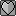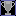Should have posted that I was already doing this, but:

HTML:
``````<h2><a name="pid_affected">More Things affected by the PID</a></h2>

<h3><a name="how_shiny">How the Game Makes a Pokemon Shiny</a></h3>

<p>Very rarely, you might encounter a Pokemon having a different coloration than usual. This is called a shiny Pokemon. The game’s criterion for making a shiny Pokemon depends in part on its PID, but also depends on two other numbers.</p>

<p>One of these numbers is visible in the game. It is called the Trainer ID. This is the number you have when you look at your Trainer card, and is a number between 0 and 65535 (between  and [FFFF] in hexadecimal). The other number is also a number between 0 and 65535 but is unfortunately invisible to you. It is called the Secret ID. Currently, there’s no way you can know your Secret ID without looking at your save file or without using cheat codes.</p>

<p>So how does the game determine that a Pokemon is shiny? Here’s how.</p>

<p>Split the PID in two 16-bit numbers. That is, if the PID was [465DB901], it would split it in two as [465D] and [B901]. We shall call the first 4 hexadecimal digits the HID (high ID) and the last 4 hexadecimal digits the LID (Low ID). Now convert the HID, LID, Trainer ID (which we shall denote by TID for short) and Secret ID (denoted by SID for short) to binary, and compare the first 13 bits of all four, like so:</p>

<pre>HID: <strong><em>x</em></strong>xxxxxxxxxxxx|xxx
LID: <strong><em>x</em></strong>xxxxxxxxxxxx|xxx
TID: <strong><em>x</em></strong>xxxxxxxxxxxx|xxx
SID: <strong><em>x</em></strong>xxxxxxxxxxxx|xxx</pre>

<p>Here we’re counting the number of 1's in the first bit of the HID, LID, TID and SID. If there are either 1 or 3 ones among those bits, the Pokemon is automatically not shiny. If there aren’t, the game counts the second bits. Again, if there are either 1 or 3 ones among those bits, the Pokemon is automatically not shiny. The game checks the bits of the HID, LID, TID and SID up to their thirteenth one, and all of them would need to have 0, 2 or 4 ones for the Pokemon to be shiny! This is a one in 8192 (2 to the power of 13) chance of happening.</p>

<p>Let’s have an example. Suppose the Trainer ID of the player is 43288, or [A918] in hexadecimal, and the Secret ID is 6075, or [17BB] in hexadecimal. Suppose also that the PID of the Pokemon is [B58F0B2A]. We split the PID in two, the HID [B58F] and the LID [0B2A], and convert the HID, LID, Trainer ID and Secret ID to binary. Thus we would have:</p>

<pre>HID: 1011010110001|111
LID: 0000101100101|010
TID: 1010100100011|000
SID: 0001011110111|011</pre>

<p>We now count the number of bits in the first bit, second bit, third bit ... up to the thirteenth bit of each of these four 16-bit numbers.</p>

<pre> HID: 1011010110001|111
LID: 0000101100101|010
TID: 1010100100011|000
SID: 0001011110111|011
Ones: 2022222420224</pre>

<p>Since counting the ones of each bit up to the thirteenth one in turn never yields a number that is not 1 or 3, the Pokemon will be shiny!</p>

<h3><a name="silcoon_cascoon">How to determine whether Wurmple evolves into Silcoon or into Cascoon</a></h3>

<p>There are many hoaxes on the internet as to how Wurmple evolves into Silcoon or into Cascoon. Some say that it is determined by gender, other say it is determined by whether or not it is night or day. The truth is that it is determined from the PID of Wurmple as follows.</p>

<p>Look at the last 16 bits of the PID. We have called it the LID in the previous section. Convert the LID to decimal, and look at the last digit of this decimal number. If this digit is 0, 1, 2, 3 or 4, Wurmple would evolve into Silcoon. If the digit is 5, 6, 7, 8, or 9, Wurmple would evolve into Cascoon.</p>

<p>Let’s provide an example. Say a Wurmple has a PID of [5CF4091C]. The last 16 bits of the PID are [091C], which is equal to 2332 in decimal. Since the last digit of this decimal number is 2, this Wumple would evolve into Silcoon.</p>

<h3><a name="unown_shape">How to determine Unown’s shape</a></h3>

<p>As claimed previously, even Unown’s shape is determined from its PID.</p>

<p>First convert the PID to binary. Look at the seventh, eighth, fifteenth, sixteenth, twenty-third, twenty-fourth, thirty-first and thirty-second bits of the PID, i.e. the ones underlined below:</p>

<pre>xxxxxx<strong><em>xx</em></strong>xxxxxx<strong><em>xx</em></strong>xxxxxx<strong><em>xx</em></strong>xxxxxx<strong><em>xx</em></strong></pre>

<pre>Combine these 8 bits together to form an 8-bit number, and convert it to decimal. If this number is 28 or more, subtract 28 from this number until it is a number between 0 and 27. This number would then correspond to the Unown symbol according to the following table:</pre>

<table class="sortable">
<tr>
<th>Number</th>
<th>Unown Shape</th>
</tr>
<tbody>
<tr><td>0</td><td>A</td></tr>
<tr class="a"><td>1</td><td>B</td></tr>
<tr><td>2</td><td>C</td></tr>
<tr class="a"><td>3</td><td>D</td></tr>
<tr><td>4</td><td>E</td></tr>
<tr class="a"><td>5</td><td>F</td></tr>
<tr><td>6</td><td>G</td></tr>
<tr class="a"><td>7</td><td>H</td></tr>
<tr><td>8</td><td>I</td></tr>
<tr class="a"><td>9</td><td>J</td></tr>
<tr><td>10</td><td>K</td></tr>
<tr class="a"><td>11</td><td>L</td></tr>
<tr><td>12</td><td>M</td></tr>
<tr class="a"><td>13</td><td>N</td></tr>
<tr><td>14</td><td>O</td></tr>
<tr class="a"><td>15</td><td>P</td></tr>
<tr><td>16</td><td>Q</td></tr>
<tr class="a"><td>17</td><td>R</td></tr>
<tr><td>18</td><td>S</td></tr>
<tr class="a"><td>19</td><td>T</td></tr>
<tr><td>20</td><td>U</td></tr>
<tr class="a"><td>21</td><td>V</td></tr>
<tr><td>22</td><td>W</td></tr>
<tr class="a"><td>23</td><td>X</td></tr>
<tr><td>24</td><td>Y</td></tr>
<tr class="a"><td>25</td><td>Z</td></tr>
<tr><td>26</td><td>?</td></tr>
<tr class="a"><td>27</td><td>!</td></tr>
</tbody>
</table>

<p>Let’s give an example. Suppose an Unown has PID [4C07DE71]. We first convert this PID to binary, getting</p>

<pre>010011<strong><em>00</em></strong>000001<strong><em>11</em></strong>110111<strong><em>10</em></strong>011100<strong><em>01</em></strong></pre>

<p>Combine the underlined bits together, getting 00111001, and convert it to decimal, getting 57. 57 is larger than 27, so it is subtracted by 28, becoming 29. 29 is again larger than 27, so it is subtracted by 28, getting 1. 1 corresponds to B, and hence the Unown would have the shape of a B.</p>

<h3><a name="spinda_spots">How Spinda’s spots are determined</a></h3>

<p>Spinda’s spots are the most useless thing ever implemented in the history of computer implementations, but, for the sake of completeness, we explain exactly how Spinda’s spots are placed on its rubbery body, because the PID, again, is the culprit.</p>

<p>Look at Spinda’s PID in hexadecimal. Convert each hexadecimal digit into decimal in turn. So, for example, if Spinda’s PID is [65492BDA], we would have 6 = 6, 5 = 5, 4 = 4, 9 = 9, 2 = 2, B = 11, D = 13 and A = 10. From these numbers, the co-ordinates (5, 6), (9, 4), (11, 2) and (10, 13) are thus constructed. These correspond to the coordinates of the upper-left hand corner of Spinda’s left face spot, its right face spot, its left ear spot and its right ear spot respectively. (Spinda’s left spots appear on the right in its front sprite and vice-versa.) All Spinda have exactly 4 spots. If some Spinda appear to have less than four spots, it is either because some spots are superimposed on each other or because the coordinate for some spots does not fall completely on Spinda’s sprite.</p>

<p>To put them in place, the game adds 18 to the x-coordinate and 19 to the y-coordinate of the left face spot. It also adds 6 to the x-coordinate and 18 to the y-coordinate of the right face spot. Lastly, it adds 24 to the x-coordinate and 1 to the y-coordinate of of the left ear spot. The right ear spot’s coordinates are left unchanged.</p>

<pre>Thus we have:</pre>

<pre> Left face spot upper-left hand coordinates: (23, 25)
Right face spot upper-left hand coordinates: (15, 22)
Left ear spot upper-left hand coordinates: (35, 3)
Right ear spot upper-left hand coordinates: (10, 13)</pre>

<h2><a name="pid_chained_shiny">The PID of Chained Shiny Pokemon</a></h2>

<p>A new feature in Diamond and Pearl is the PokeRadar, which allows you to chain Pokemon. Chained Pokemon, besides having the advantage of always catching Pokemon of the same species, have a greater probability for them to appear shiny. This section explains in detail how the game determines whether or not a chained Pokemon is shiny, and how it constructs its PID so that it conforms to the criterion that appears in the previous section entitled ‘How the game makes a Pokemon shiny’.</p>

<h3><a name="probability_appear_shiny">The Probability of a Chained Pokemon to appear Shiny</a></h3>

<p>This depends on the chain number you’ve attained, which cannot be greater than 40. Suppose your chain number is c. The probability that a chained Pokemon is shiny is governed by the following formula:</p>

<pre>Probability = floor((14747 – 40 × c) ÷ (1640 – 40 × c)) ÷ 65536</pre>

<p>where floor(x) is x rounded down.</p>

<p>Hereunder is the probability of catching a shiny Pokemon knowing your chain length:</p>

<table class="sortable">
<tr>
<th>Chain Length</th>
<th>Probability of catching a Shiny Pokemon</th>
</tr>
<tbody>
<tr><td>0</td><td>1 in 8192</td></tr>
<tr class="a"><td>1</td><td>1 in 7282</td></tr>
<tr><td>2</td><td>1 in 7282</td></tr>
<tr class="a"><td>3</td><td>1 in 7282</td></tr>
<tr><td>4</td><td>1 in 7282</td></tr>
<tr class="a"><td>5</td><td>1 in 6554</td></tr>
<tr><td>6</td><td>1 in 6554</td></tr>
<tr class="a"><td>7</td><td>1 in 6554</td></tr>
<tr><td>8</td><td>1 in 6554</td></tr>
<tr class="a"><td>9</td><td>1 in 5958</td></tr>
<tr><td>10</td><td>1 in 5958</td></tr>
<tr class="a"><td>11</td><td>1 in 5958</td></tr>
<tr><td>12</td><td>1 in 5461</td></tr>
<tr class="a"><td>13</td><td>1 in 5461</td></tr>
<tr><td>14</td><td>1 in 5041</td></tr>
<tr class="a"><td>15</td><td>1 in 5041</td></tr>
<tr><td>16</td><td>1 in 4681</td></tr>
<tr class="a"><td>17</td><td>1 in 4681</td></tr>
<tr><td>18</td><td>1 in 4369</td></tr>
<tr class="a"><td>19</td><td>1 in 4369</td></tr>
<tr><td>20</td><td>1 in 4096</td></tr>
<tr class="a"><td>21</td><td>1 in 3855</td></tr>
<tr><td>22</td><td>1 in 3641</td></tr>
<tr class="a"><td>23</td><td>1 in 3449</td></tr>
<tr><td>24</td><td>1 in 3277</td></tr>
<tr class="a"><td>25</td><td>1 in 3121</td></tr>
<tr><td>26</td><td>1 in 2979</td></tr>
<tr class="a"><td>27</td><td>1 in 2731</td></tr>
<tr><td>28</td><td>1 in 2521</td></tr>
<tr class="a"><td>29</td><td>1 in 2341</td></tr>
<tr><td>30</td><td>1 in 2185</td></tr>
<tr class="a"><td>31</td><td>1 in 1986</td></tr>
<tr><td>32</td><td>1 in 1771</td></tr>
<tr class="a"><td>33</td><td>1 in 1598</td></tr>
<tr><td>34</td><td>1 in 1394</td></tr>
<tr class="a"><td>35</td><td>1 in 1192</td></tr>
<tr><td>36</td><td>1 in 993</td></tr>
<tr class="a"><td>37</td><td>1 in 799</td></tr>
<tr><td>38</td><td>1 in 596</td></tr>
<tr class="a"><td>39</td><td>1 in 400</td></tr>
<tr><td>40+</td><td>1 in 200</td></tr>
</tbody>
</table>

<p>Notice that the probabilities are not very good unless your chain’s length becomes 30 or more, so aim for as long a chain as possible.</p>

<h3><a name="pid_iv_creation_shiny">How the PID and IVs of a Chained Shiny Pokemon is created</a></h3>

<p>First, the game calls the RNG twice to create the PID as usual. Remember that the first number generated would be the LID (last 16 bits) of the PID, while the second one would be the HID (first 16 bits) of the PID. The game now needs to ‘fix’ the PID such that the criterion for the Pokemon to appear shiny is attained. This is done as follows.</p>

<p>Convert the Trainer ID and Secret ID to binary, and look at their thirtheenth, twelfth, eleventh, etc. bits of both, until we reach their first bit. Suppose we’re looking at the i’th bit. For each of these pairs of bits, the RNG is called once and the resulting random number’s last bit is noted.</p>

<p>If the pair of bits at the i’th position of TID and SID are the same (i.e. either both are 0 or both are 1), the HID and LID’s i’th bits are both set to the last bit of the random number generated. If the pair of bits at the i’th position of TID and SID are different (i.e. one is 0 and the other one is 1), the LID’s i’th bit is set to the last bit of the random number generated, and the HID’s i’th bit is set to the reverse bit (1 if it was 0, 0 if it was 1) of the last bit of the random number generated.</p>

<p>Following this procedure, this would ensure that the number of 1’s in the i’th position of the TID, SID, HID and LID is either 0, 2 or 4. Since this would be true for all bits between the first and the thirteenth, the Pokemon would be ‘forced’ to appear shiny.</p>

<p>After this is done, there are two subsequent calls to the RNG to create the IVs as usual. Thus, the RNG is called 17 times in all to generate a chained shiny Pokemon: twice to generate the initial PID, 13 times to ‘fix’ the PID, and twice to generate the IVs.</p>

<h3><a name="example_generation_shiny">An Example of generating the PID of a Chained Shiny Pokemon</a></h3>

<p>Suppose the player encounters a chained shiny Pokemon. We suppose the player has Trainer ID (TID) 38710 and Secret ID (SID) 13099. We also suppose that the current RNG seed is [69AC550F]. We call the RNG twice, getting the numbers [18E5] and [1DD6]. Hence, the LID is [18E5] and the HID is [1DD6]. The numbers in binary are:</p>

<pre>TID: 1001011100110110
SID: 0011001100101011
HID: 0001110111010110
LID: 0001100011100101</pre>

<p>We now need to fix the HID and LID such that the Pokemon is shiny. We start looking from the thirteenth bit.</p>

<p>We call the RNG, getting [A013]. When converted to binary, its last digit is 1. (To know at a glance if the last bit of a hexadecimal number is 0 or 1, look at the last hexadecimal digit. If it is 0, 2, 4, 6, 8, A, C or E, the last bit would be 0, otherwise it would be 1.)</p>

<p>The TID and SID’s thirteenth bits are 0 and 1 respectively, hence they are different. Thus, we make the thirteenth bit of LID as 1 (last digit of the last random number generated) and the first bit of HID as 0. So we have:</p>

<pre>TID: 1001011100110110
SID: 0011001100101011
HID: 000111011101<strong><em>0</em></strong>110
LID: 000110001110<strong><em>1</em></strong>101</pre>

<p>Next we go to the twelfth bit. We generate another random number, getting [A4AE], whose last bit is 0. TID’s and SID’s twelfth bits are 1 and 0 respectively. Hence the twelfth bit of the LID is made to be 0 and the HID's twelfth bit is made to be 1.</p>

<pre>TID: 1001011100110110
SID: 0011001100101011
HID: 00011101111<strong><em>10</em></strong>110
LID: 00011000111<strong><em>01</em></strong>101</pre>

<p>Moving on to the eleventh bit, we call the RNG again, getting [77AD] whose last bit is 1. The TID and SID’s eleventh bit are both 1. Thus we make both the HID and the LID's eleventh bits to be 1.</p>

<pre>TID: 1001011100110110
SID: 0011001100101011
HID: 0001110111<strong><em>110</em></strong>110
LID: 0001100011<strong><em>101</em></strong>101</pre>

<p>For the tenth bit, we again call the RNG, getting [B237] whose last bit is 1. The TID and SID’s tenth bits are both 0. Thus we make the fourth bit of LID and HID both 1.</p>

<pre>TID: 1001011100110110
SID: 0011001100101011
HID: 000111011<strong><em>1010</em></strong>110
LID: 000110001<strong><em>1101</em></strong>101</pre>

<p>Continuing doing this until we reach the first bit, we get:</p>

<pre> TID: 1001011100110110
SID: 0011001100101011
HID: <strong><em>1100001101110</em></strong>110
LID: <strong><em>0110011101101</em></strong>101
Ones: 2222024402422</pre>

<p>The last bit of each of the subsequent random numbers generated was 0, 1, 1, 1, 0, 0, 1, 1, 0 for the ninth, eighth, seventh, ... first bit respectively. As you can see, the HID and LID now satisfy the criterion for the Pokemon to appear shiny.</p>

<p>Thus the PID of the chained shiny Pokemon would be</p>

<pre>11000011011101100110011101101101</pre>

<p>which is equivalent to [C376676D] in hexadecimal, or 3279316845 in decimal. Two subsequent RNG calls would then be made to create the Pokemon’s IVs normally.</p>``````